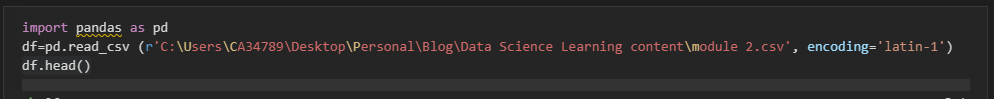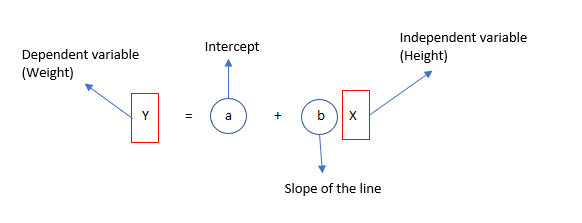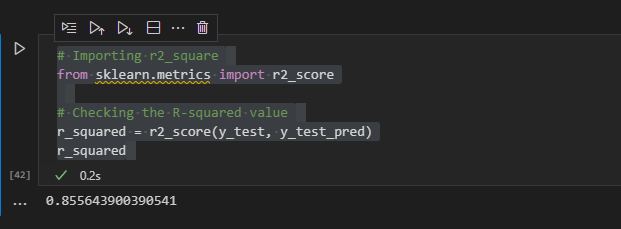# Module 2 - Process

### Data Science Project​

Typically, before starting any data science project, we need data to perform analysis and build a model. Though you will need first to understand the business need and collect the data in the real world, we are providing the data for you in this module. Please use this dataset for the next steps.### 1. Importing the Dataset​

Let us import the data set and load it onto the Jupyter notebook. You can use the online python editor available on the learning content page.### 2. Reviewing the Dataset​

We can review the dataset by looking at the first few rows of data. You can do that by using the head function in python. It helps provide an idea about the data structure.## Exploratory data analysis​

Before building any model, it is essential to understand the data set. EDA (exploratory data analysis) is a crucial step in building any successful model. The dataset used for this model is straightforward. We are trying to predict the weight of the individual based on their height. So let us first see if there is any correlation between height and weight of the individual.

### Descriptive statistics –​

Run sns heatmap plot to check for correlation between dependent and independent variables.

Dependent variable: Weight Independent variable: HeightHeatmap is a two-dimensional visual representation of the variables. It is considered one of the best visual graphical representations when you want to show complex data. It is comprised of square boxes, and the x-axis and y-axis represent the variables. For correlation, each square box represents the level of correlation between the variable on the X-axis Vs. variable on the Y-axis. The annotations shown in each square box represent the correlation value. If you look across the matrix, the correlation value for weight and height is 0.92, which indicates that these variables are highly correlated. Please note that if you look diagonally, the values will always be one as you are just comparing the variable with itself.

Regarding correlation:

• Values closer to 1 show a high correlation, and values closer to 0 show the lowest correlation.
• A positive correlation value shows that the variables are positively correlated. For example, taller individuals usually weigh more.
• A negative correlation value shows that the variables are negatively correlated.

Plot sns pair plot to visually represent the positive/negative correlation between the variables.So, based on the sns pair plot, the Height is positively correlated to Weight of the individual. Now, let's build a model that will predict the individual's weight based on height. Below are the steps to follow to create a model.

### Create, Train and Test datasets​We are first splitting the dataset into train and test data sets. We will build the model based on the train data set and test it on the test dataset.

### Build a simple Linear Regression Model​

Now, we are ready to build a simple linear regression model. Remember, the regression model predicts the continuous variables only. Like weight, sales, the number of births, etc. So, we should choose a regression model only when we have continuous dependent and independent variables.

How do we build the model? What packages do we need? We will be using existing python packages to build the model.

Below are the packages:

• Sklearn
• Statsmodel

Below is the equation for simple linear regression:### Add intercept to the model​

The Statsmodel library that we will be using for building the linear regression model will fit a line for our dataset. Now, let's input the intercept value using the Statsmodel library.### Fit the regression line​

Now, let’s fit the regression line using the stats model.## Understand the results​

• R-squared value is 0.855. This shows that 85.5% of the variance in the weight can be explained by height.
• F statistic is pretty low, which shows that the model fit is statistically significant.
• The coefficient for height is 7.7 and P-value is close to 0. This shows that the coefficient is statistically significant.

Based on the summary, we can now derive the linear regression equation using intercept and slope.

Weight = -351.4621 + 7.7275 * height

Plot the regression line using the equation:### Now predict the weight based on the height in the test dataset​### What is r to the power of 2?​Our model still shows that the height can explain 85% of the variance in the weight for the test dataset. This score aligns with the training model r2. This indicates that our model is pretty stable even if we use a new dataset.

In this module, we have learned how to load the dataset and perform simple exploratory data analysis. For a given dataset, if we have a continuous dependent and independent variable, a simple linear regression statistical model can be used to predict the dependent variable. In our next module, we will learn more about what residuals are, how to derive them based on our model, and the importance of residuals in building a model.